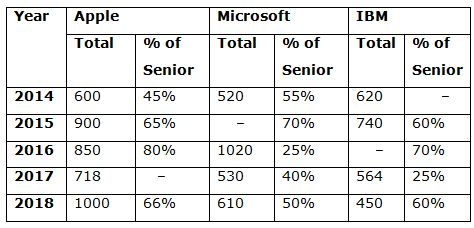# NIACL AO Mains– Quantitative Aptitude Questions Day- 22

Dear Readers, Bank Exam Race for the Year 2019 is already started, To enrich your preparation here we have providing new series of Practice Questions on Quantitative Aptitude – Section. Candidates those who are preparing for NIACL AO Mains 2019 Exams can practice these questions daily and make your preparation effective.

[WpProQuiz 5302]

Directions (Q. 1 – 5): What value should come in the place of question mark (?) in the following questions?

1) 18 % of 750 + (5/13) of 16250 – 6 % of 800 =?

a) 5549

b) 5176

c) 6337

d) 6893

e) None of these

2) ? % of 400 = 262 + (422 – 192) × 33 ÷ 30.5

a) 445

b) 385

c) 560

d) 620

e) None of these

3) (5274 + 1442) ÷ 23 + 492 + (2/9) of 3564 – 52 =?

a) 3156

b) 3433

c) 3952

d) 2880

e) None of these

4) 17280 ÷ 12 + 44 × 36 + 452 =? + √23104

a) 3562

b) 4378

c) 5156

d) 4897

e) None of these

5) (5/3) of 1827 + 32 % of 4450 + (13/8) ÷ (9/72) =?

a) 5358

b) 3895

c) 4482

d) 6123

e) None of these

Directions (Q. 6 – 10): Study the following information carefully and answer the given questions:

The following table shows the total number of employees in 3 different companies in 5 different years and the percentage of senior employees among them. Some values are missing here.6) The total number of employees from Microsoft increased by 50% from 2014 to 2015 then find the number of senior employees in 2015 in the same Company?

a) 251

b) 546

c) 598

d) 472

e) None of these

7) The number of senior employees from IBM in 2016 is 490, then find the ratio of Senior employees and other employees in the same year in IBM?

a) 7: 3

b) 5: 9

c) 6: 3

d) 9: 5

e) None of these

8) If the senior employees in 2017 in all the Companies together is 712, then the number of senior employees in Company Apple is what percentage of total number of employees in Apple?

a) 50%

b) 25%

c) 35%

d) 40%

e) None of these

9) Find the total number of senior employees from Microsoft in all the years together, if the total number of employees from Microsoft in 2015 is 700?

a) 1172

b) 1375

c) 1548

d) 1954

e) None of these

10) What is the difference between the number of senior employees in 2018 and 2016 in all the Companies together, if the total number of employees from IBM in 2016 is 700?

a) 180

b) 120

c) 155

d) 140

e) None of these

Directions (1-5):

(18/100)*750 + (5/13)*16250 – (6/100)*800 = x

X = 135 + 6250 – 48 = 6337

(x/100)*400 = 262 + [(42 + 19) (42 – 19)]*(33/30.5)

4x = 262 + (61*23*33/30.5)

4x = 262 + 1518 = 1780

X = 1780/4 = 445

6716/23 + 492 + (2/9)*3564 – 52 = x

X = 292 + 2401 + 792 – 52

X = 3433

(17280/12) + (44*36) + 2025 – 152 = x

X = 1440 + 1584 + 2025 – 152 = 4897

(5/3)*1827 + (32/100)*4450 + (13/8)*(72/9) = x

X = 3045 + 1424 + 13 = 4482

Directions (6-10):

Total number of employees from Microsoft in 2015,

520*(150/100) = 780

Senior employees in Microsoft = 780*(70/100) = 546

The number of senior employees from IBM in 2016 is 490,

70% of total employees = 490

Total employees = 700

The ratio of Senior employees and other employees in the same year in IBM,

= > 490: 210

= > 7: 3

718*(x/100) + 530*(40/100) + 564*(25/100) = 712

718*(x/100) + 212 +141 = 712

718*x/100 = 359

X = 359*(100/718) = 50%

Required total

= > [520*(55/100) + 700*(70/100) + 1020*(25/100) + 530*(40/100) + 610*(50/100)]

= > (286 + 490 + 255 + 212 + 305)

= > 1548

The number of senior employees in 2016

= > 850*(80/100) + 1020*(25/100) + 700*(70/100)

= > 680 + 255 + 490 = 1425

The number of senior employees in 2018

= > 1000*(66/100) + 610*(50/100) + 450*(60/100)

= > 660 + 305 + 270 = 1235

Required difference = 1425 – 1235 =190